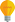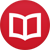# Applying an operator with respect to a variable

by Jakub MarianTip: See my list of theMost Common Mistakes in English. It will teach you how to avoid mis­takes with com­mas, pre­pos­i­tions, ir­reg­u­lar verbs, and much more.

There are seemingly two kinds of “operations” used in mathematics when it comes to working with functions; you can either take a function $f$ and apply an operator, say $A$, to it (which would be usually written as $Af$ or $A(f)$), or you can take an expression of the form $f(x)$ and change it according to some rules (such as $÷{d}{dx}(x^3) = 3x^2$.

The first notation, $Af$ or $A(f)$, is actually the easier one to grasp from the mathematical viewpoint. We are used to applying functions like $\cos(π) = -1$; that is, the function $\cos$ gets $π$ as the input and returns $-1$ as the output. In the very same way, an operator is just something that gets a function as the input and returns a number or another function as the output. For example, if $V_a(f) = f(a)$, i.e. $V_a$ applied to a function is the value of this function at $a$, then $V_π(\cos) = -1$, $V_{π/2}(\sin) = 1$, etc.

What do expressions like $\lim_{x→a} f(x)$ mean, then? We can certainly use them to define an operator, e.g. $\mathrm{Zero}(f) = \lim_{x→0} f(x)$, but in fact, we can think about $\lim$ as already being an operator in the following mannerlet’s define:

$$\underset{x}{A} \,something = A(x ↦ something)\quad\text{ resp. }\quad\underset{x}{A}\, f(x) = A(f)$$

(We would write $A_x$ inside of a block of text.) For example:

$$\underset{x}{\mathrm{Zero}}\, ÷{\tan(x)}{\sin(x)} = \mathrm{Zero}\l(÷{\tan}{\sin}\r) = \lim_{x→0} ÷{\tan(x)}{\sin(x)} = 1\,.$$

Similarly, we could write

$$\underset{x\,}{V_π}\,\,\, x\cos(x) = -π\,,$$

but this is not very natural, is it; the $x$ looks strange just floating around. Wouldn’t it be much more natural to write

$$\underset{x=π}{V}\,\,\, x\cos(x) = -π$$

instead? It means “the value of $x\cos(x)$ for $x = π$”, after all. We can think in the very same manner about the operator of limit. Let’s define

$$\textstyle\lim_a f = \text{the limit of f at a}$$

(Of course, it would be possible to define “the limit of $f$ at $a$” using mathematical symbols, but let’s just leave it like that for simplicity.) Now, we have an operator, and we can write expressions like

$$\textstyle\lim_0(\cos) = 1\,.$$

The natural way to denote variables for the operator $\lim_a$ is $\lim_{x→a}$, for example: $$\lim_{x→0}\, ÷{\tan(x)}{\sin(x)} = {\textstyle \lim_0} \l(÷{\tan}{\sin}\r) = 1\,.$$

The second term reveals the true mathematical meaning of the process, while the first term is just a different way to denote it.

You may have noticed that I deliberately avoided the functions $x^n$ because there is no standard way to denote them without using variables. There are two obvious solutions: We can either assign a new symbol to it, say $\id^n$ (where $\id(x) = x$), or we can assign another meaning to x^n.

Personally, I prefer the symbol $\id$ because expressions like $\lim_π (x\cos) = -π$ appear “optically incomplete” to me, but it is clear that the parameter of $\lim_π (\id\cos)$ is a function, not an expression containing a variable.

This new symbol, $\id$, is mightier than it might seem at first. We can actually denote any function using it as we would be able to using variables. For example, we can write $e^\id$ instead of $e^x$, $\id^2$ instead of $x^2$ and so on. The major advantage of expressions with $\id$ is that they already represent functions, so it is all right to write not only expressions like

$$\id^2(3) = 9, \quad \sin(2\id) = 2\sin\cos,\quad {\textstyle\lim_0} ÷{\sin}{\id} = 1$$

but also compositions like

$$(\id^2+e^\id) ∘ (\sin + \cos) = (\sin + \cos)^2 + e^{(\sin + \cos)}\,.$$

The concept of applying an operator with respect to a variable can appear in unexpected places. Have you ever realized that there is an operator of sum? We can define:

$$∑_{m}^n f = f(m) + f(m+1) + … + f(n)$$

The usual way we write sums is just a way to write variables for this operator. There are certain advantages of not using variables in certain types of computations with sums, namely when working with indefinite sums. Indefinite sum is the equivalent of indefinite integration in the discrete case, and there is not really any standard convenient notation for it.

Both indefinite sums and indefinite integration can be formalized very naturally as the inverse operators to finite differences and differentiation, respectively. Using this approach, it is possible, for example, to write the fundamental theorem of calculus as

$$∫ f(g)g’ = \l(∫f\r)(g)\,,$$

but more on that later.

The situation with the operators $÷{d}{dx}$ and $÷{∂}{∂x}$ is somewhat more complicated because they are used in two different meanings. Let $D$ be the operator of taking the first derivative, i.e. $D(f) = f’$. The traditional symbol $÷{d}{dx}$ can mean the $D$ itself, i.e. both $÷{d}{dx} f = Df$, or it can mean something like “$D_x f(x)$”.

The notation $÷{d}{dx} f(x)$ introduces a new concept. It means $÷{d}{dx} f(x) = (Df)(x)$, which could be defined as

$$÷{d}{dx}(something) = \l(D(x ↦ something)\r)(x)\,,$$

i.e. basically the same as the definition above, but since the result is a function, not a number, we introduce the variable again on the right-hand side.

By the way, I have written several educational ebooks. If you get a copy, you can learn new things and support this website at the same time—why don’t you check them out?